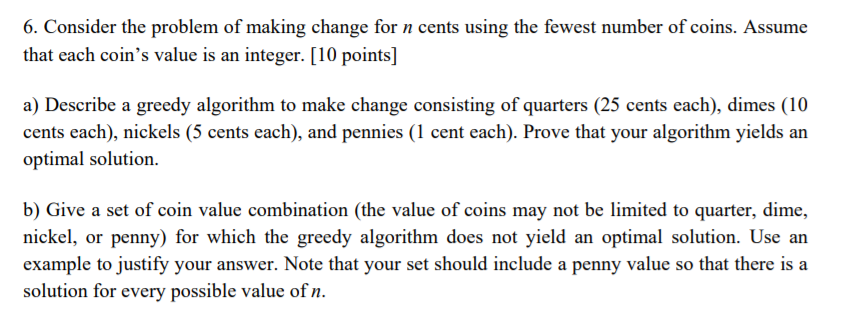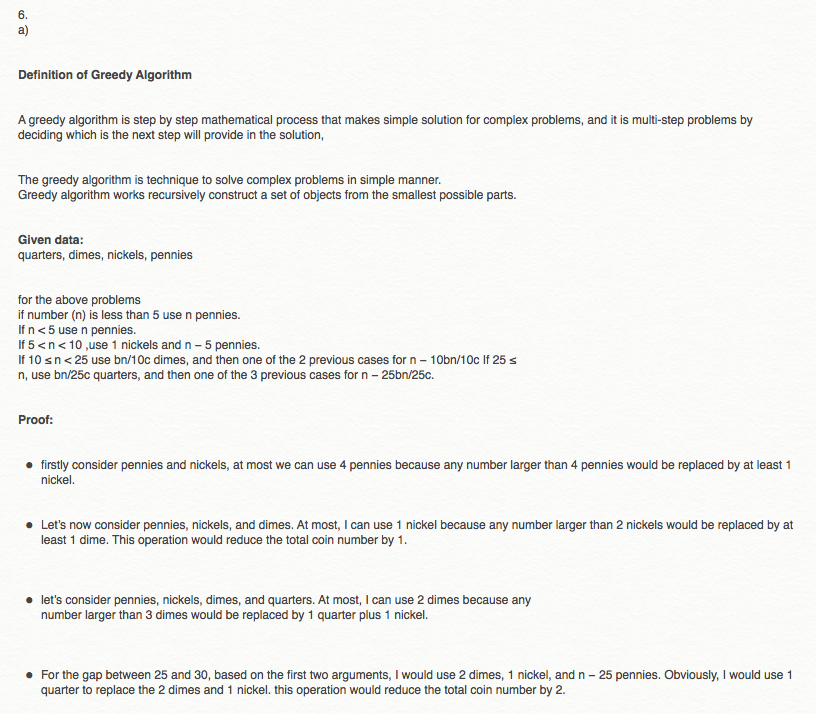# Question & Answer: Consider the problem of making change for n cents using the fewest number of coins. Assume that each coin's value is…..Consider the problem of making change for n cents using the fewest number of coins. Assume that each coin’s value is an integer. a) Describe a greedy algorithm to make change consisting of quarters (25 cents each), dimes (10 cents each), nickels (5 cents each), and pennies (1 cent each). Prove that your algorithm yields an optimal solution. b) Give a set of coin value combination (the value of coins may not be limited to quarter, dime, nickel, or penny) for which the greedy algorithm does not yield an optimal solution. Use an example to justify your answer. Note that your set should include a penny value so that there is a solution for every possible value of n.